#### You may also like### Fixing It

A and B are two fixed points on a circle and RS is a variable diamater. What is the locus of the intersection P of AR and BS?### OK! Now Prove It

Make a conjecture about the sum of the squares of the odd positive integers. Can you prove it?### Polycircles

Show that for any triangle it is always possible to construct 3 touching circles with centres at the vertices. Is it possible to construct touching circles centred at the vertices of any polygon?

##### Age 14 to 18Challenge Level

Clara from Herlufsholm School in Denmark sent in some observations:
I noticed that the quadrilaterals formed by joining the midpoints always has two pairs of parallel sides. This means that the shape formed is always a square, rectangle or parallelogram. There didn't seem to be any counter-examples to this. This is because when you move one point (For example C) and keep all the other points the same, two points of the new shape move (R and S). This means that the top and bottom edges do not change angle (RS and QP). The other two sides however move but with the same amount and therefore also stay parallel.

PQRS always has half of the area of ABCD. One way to show that this is always true could be to draw a line through the points SQ and RP as in the picture attached. When you take a quarter of the whole image there is another quadrilateral with a line through the middle (as shown in the picture underneath figure 1).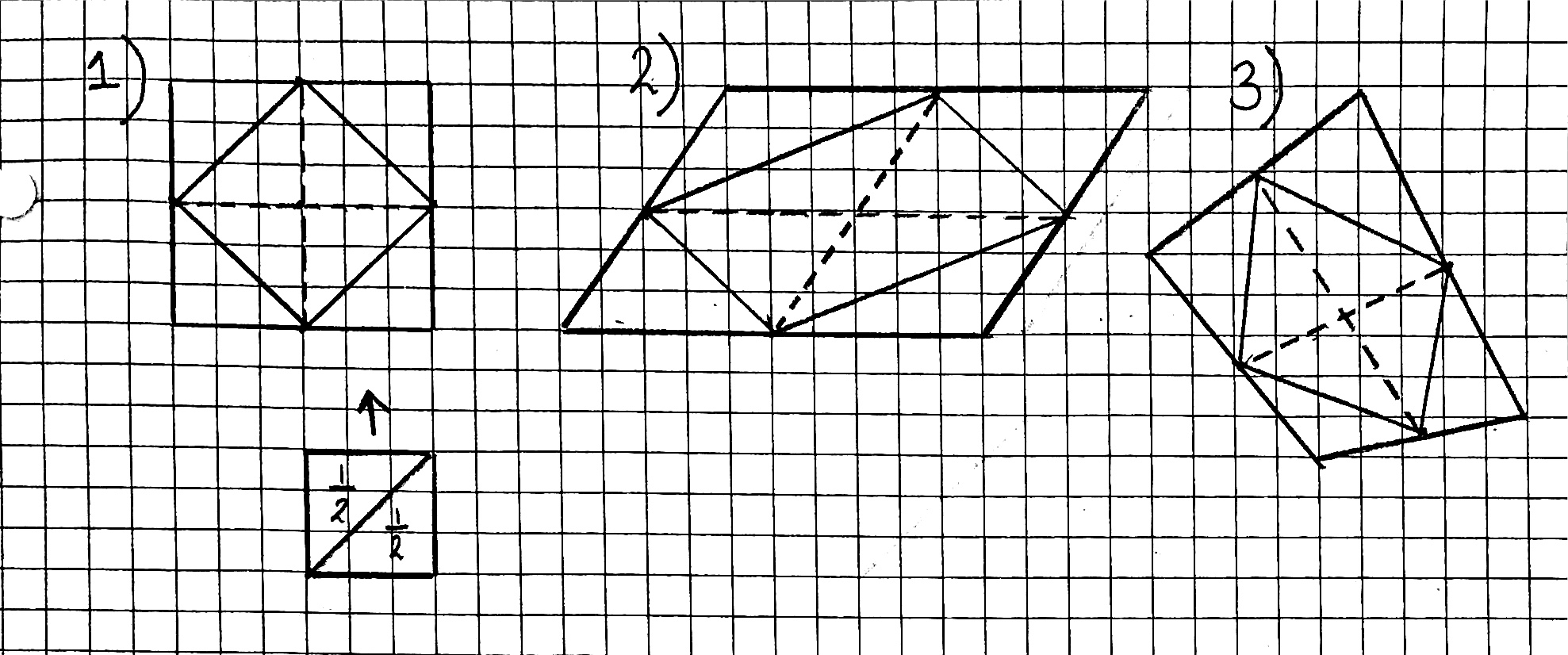If figure ABCD is concave, the ration of the area of ABCD and PQRS is still 2:1.

Clara's observations and predictions are correct, but this way of showing that PQRS has half the area of ABCD won't always work. For example, in Clara's picture 3, the triangles in the pieces are not the same size. This is shown below. In the image on the right, the yellow triangle has been rotated to show that it has a smaller area than the red triangle.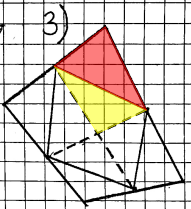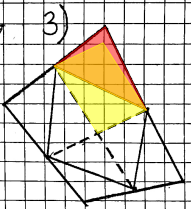Samvit from Mason Middle School in the USA sent this rigorous proof:
The new quadrilateral will always be a parallelogram.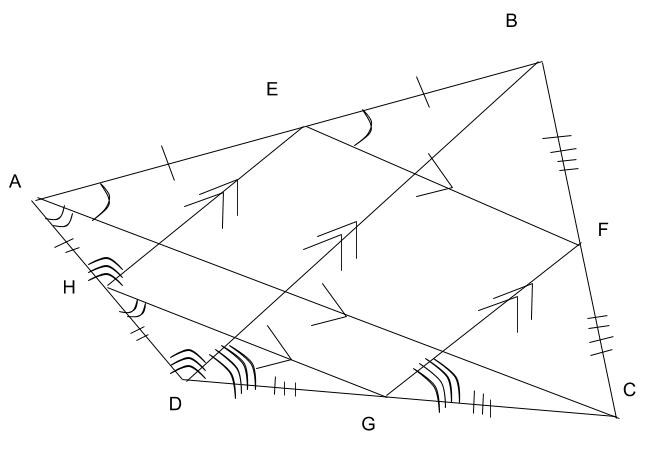For this proof, CASTC means Corresponding Angles of Similar Triangles are Congruent, which is part of the definition of a similar triangle (like CPCTC).

Take this arbitrary image of a quadrilateral, where
E is the midpoint of segment AB.
F is the midpoint of segment BC.
G is the midpoint of segment CD.
H is the midpoint of segment DA.

Two column proof:
Statement Reason
E is the midpoint of segment AB Given
segment AE is congruent to segment EB Definition of a midpoint
AE = EB Definition of congruence
AE + EB = AB segment addition postulate
EB + EB = AB Substitution
2(EB) = AB Simplify
angle EBF is congruent to angle ABC Reflexive property
F is the midpoint of BC Given
segment FC is congruent to segment BF Definition of a midpoint
FC = BF Definition of congruence
FC + BF = BC Segment addition postulate
BF + BF = BC Substitution
2(BF) = BC Simplify
triangle EBF is similar to triangle ABC SAS similarity
angle CAB is congruent to angle FEB CASTC
Segment AC is parallel to segment EF Converse of Corresponding Angles
G is the midpoint of segment CD Given
Segment GC is congruent to segment GD Definition of a midpoint
GC = GD Definition of congruence
GC + GD = CD segment addition postulate
GD + GD = CD Substitution
2(GD) = CD Simplify
angle CDA is congruent to angle GDH Reflexive property
H is the midpoint of DA Given
segment AH is congruent to segment DH Definition of a midpoint
AH = DH Definition of congruence
AH + DH = DA Segment addition postulate
DH + DH = DA Substitution
2(DH) = DA Simplify
triangle CDA is similar to triangle GDH SAS similarity
angle DAC is congruent to angle DHG CASTC
Segment AC is parallel to segment HG  Converse of Corresponding Angles
Segment EF is parallel to segment HG Transitive property of parallel lines
AE + EB = AB Segment Addition postulate
AE + AE = AB substitution
2(AE) = AB Simplify
Angle HAE is congruent to angle DAB Reflexive property
AH + DH = DA Segment Addition postulate
AH + AH = DA Substitution
2(AH) = DA Simplify
Triangle HAE is similar to triangle DAB SAS similarity
Angle AHE is congruent to angle ADB CASTC
Segment DB is parallel to segment HE Converse of Corresponding Angles
FC + BF = BC Segment Addition Postulate
FC + FC = BC Substitution
2(FC) = BC Simplify
Angle FCG is congruent to angle BCD Reflexive
GC + GD = CD Segment Addition Postulate
GC + GC = CD Substitution
2(GC) = CD Simplify
Triangle FCG is similar to triangle BCD SAS Similarity
Angle CGF is congruent to angle CDB CASTC
Segment DB is parallel to segment GF Converse of Corresponding Angles
Segment HE is parallel to segment GF Transitive property of parallel lines
EFGH is a parallelogram Definition of a parallelogram (opposite sides are parallel)

Nayanika from the Tiffin Girls' School in the UK proved that the area of the small quadrilateral is half the area of the large quadrilateral. Nayanika's proof uses the fact that the small quadrilateral is a parallelogram (although at one point she writes 'rectangle', the proof works for any parallelogram). This is Nayanika's work: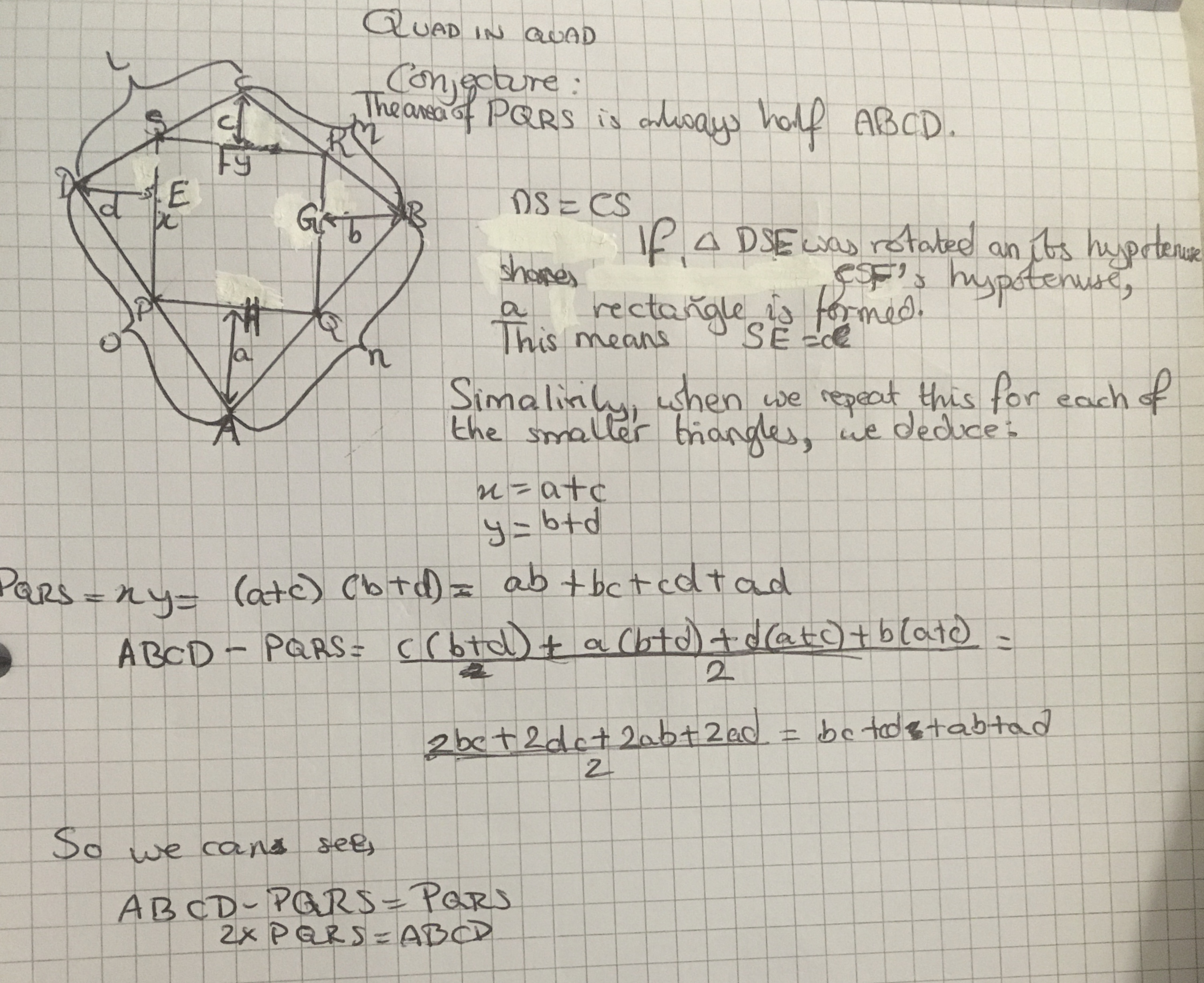Vid from High School Srednja Å¡ola ÄŒrnomelj in Slovenia used a shorter method to prove that the shape is a parallelogram, and also proved the result about the areas. Click here to see Vid's work.

Marmik from India proved that the area of PQRS is half of the area of ABCD using the formula for the area of a triangle, $\frac12ab\sin{C}$. Click here to see Marmik's work.

Ana used coordinate geometry to derive the two results. Click here to see Ana's work.